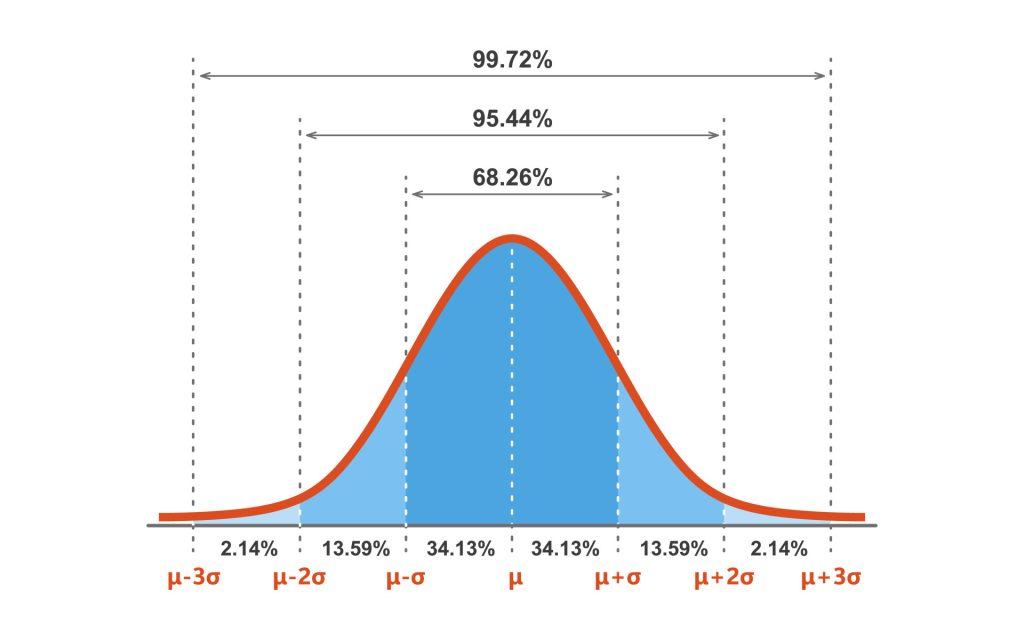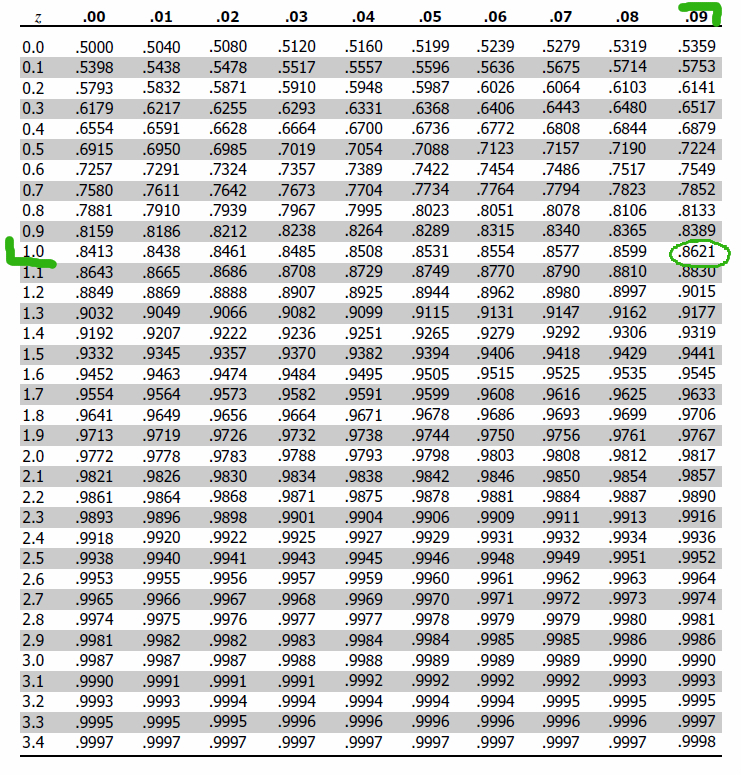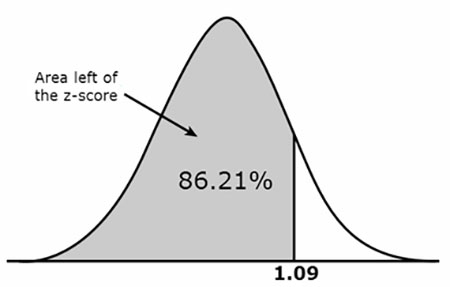# How to Use the Z-Score Table (Standard Normal Table)

A z-table, also called the standard normal table, is a mathematical table that allows us to know the percentage of values below (to the left) a z-score in a standard normal distribution (SND).Figure 1. A standard normal distribution (SND).

A z-score, also known as a standard score, indicates the number of standard deviations a raw score lays above or below the mean.

When the mean of the z-score is calculated, it is always 0, and the standard deviation (variance) is always in increments of 1.

## Left of a positive z-score

A z-score table shows the percentage of values (usually a decimal figure) to the left of a given z-score on a standard normal distribution.

For example, imagine our Z-score value is 1.09. First, look at the left side column of the z-table to find the value corresponding to one decimal place of the z-score (e.g., whole number and the first digit after the decimal point).

In this case, it is 1.0. Then, we look up the remaining number across the table (on the top) which is 0.09 in our example.Figure 2. Using a z-score table to calculate the proportion (%) of the SND to the left of the z-score.

The corresponding area is 0.8621, which translates into 86.21% of the standard normal distribution being below (or to the left) of the z-score.Figure 3. The proportion (%) of the SND to the left of the z-score.

## Right of a positive z-score

To find the area to the right of a positive z-score, begin by reading off the area in the standard normal distribution table.

Since the total area under the bell curve is 1 (as a decimal value which is equivalent to 100%), we subtract the area from the table from 1.

For example, the area to the left of z = 1.09 is given in the table as .8621. Thus the area to the right of z = 1.09 is 1 – .8621. = .1379.

## Left of a negative z-score

If you have a negative z-score, simply use the same table but disregard the negative sign, then subtract the area from the table from 1.

## Right of a negative z-score

If you have a negative z-score, simply use the same table but disregard the negative sign to find the area above your z-score.

## Finding the area between two z-scores

To find the area between two negative z-scores, we must first find the area (proportion of the SND) to the left of the lowest z-score value and the area (proportion of the SND) to the right of the highest z-score value.

Next, we must add these proportional values together and subtract them from 1 (the total area of the SND.Olivia Guy-Evans

BSc (Hons), Psychology, MSc, Psychology of Education

Associate Editor for Simply Psychology

Olivia Guy-Evans is a writer and associate editor for Simply Psychology. She has previously worked in healthcare and educational sectors.Saul Mcleod, PhD

Educator, Researcher

BSc (Hons) Psychology, MRes, PhD, University of Manchester

Saul Mcleod, Ph.D., is a qualified psychology teacher with over 18 years experience of working in further and higher education.